# assignment 1a-d in Python. please explain your steps, thanks in advance. This is the only information...

assignment 1a-d in Python. please explain your steps, thanks in advance. This is the only information that we got for this practice exercise, my teacher said that we should try to get the output literally as in the example.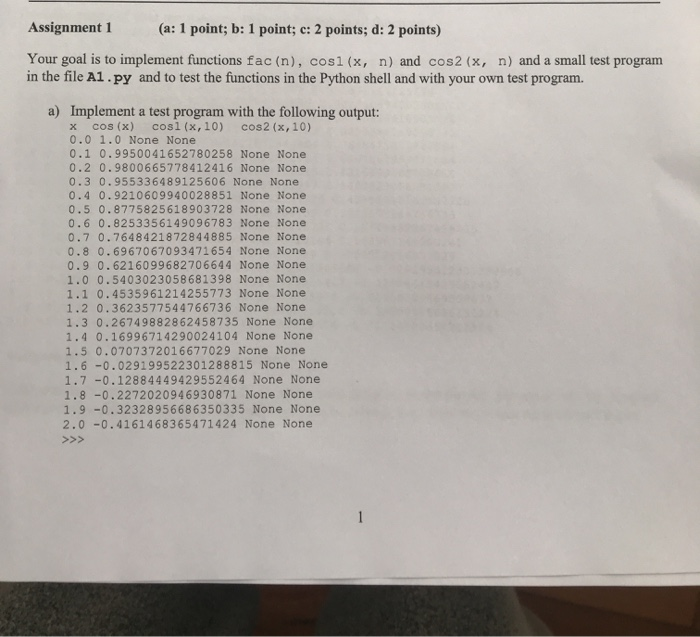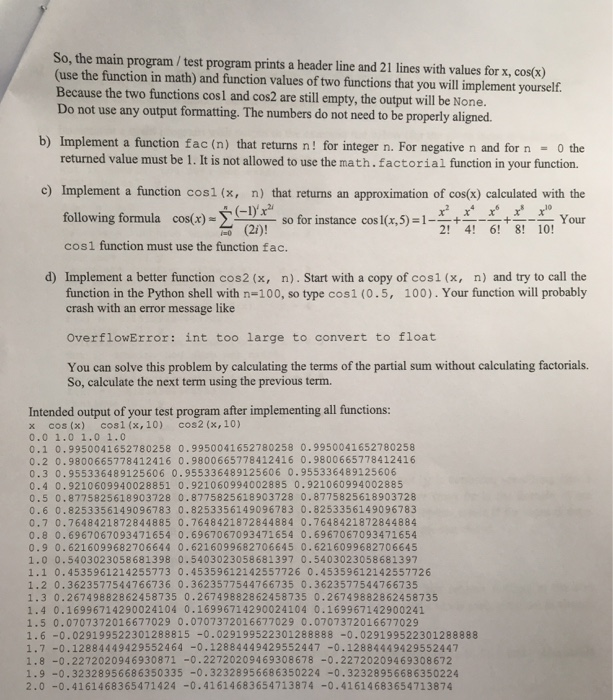Assignment 1 (a: 1 point; b: 1 point; c: 2 points; d: 2 points) Your goal is to implement functions fac(n), cos1(x, n) and cos2 (x, n) and a small test program in the file A1.py and to test the functions in the Python shell and with your own test program. a) Implement a test program with the following output: x cos(x) cos(x,10) cos2 (x,10) 0.0 1.0 None None 0.1 0.9950041652780258 None None 0.2 0.9800665778412416 None None 0.3 0.955336489125606 None None 0.4 0.9210609940028851 None None 0.5 0.8775825618903728 None None 0.6 0.8253356149096783 None None 0.7 0.7648421872844885 None None 0.8 0.6967067093471654 None None 0.9 0.6216099682706644 None None 1.0 0.5403023058681398 None None 1.1 0.4535961214255773 None None 1.2 0.3623577544766736 None None 1.3 0.26749882862458735 None None 1.4 0.16996714290024104 None None 1.5 0.0707372016677029 None None 1.6 -0.029199522301288815 None None 1.7 -0.12884449429552464 None None 1.8 -0.2272020946930871 None None 1.9 -0.32328956686350335 None None 2.0 -0.4161468365471424 None None >>>
So, the main program / test program prints a header line and 21 lines with values for x, cos(x) (use the function in math) and function values of two functions that you will implement yourself. Because the two functions cosl and cos2 are still empty, the output will be None. Do not use any output formatting. The numbers do not need to be properly aligned. b) Implement a function fac (n) that returns n! for integer n. For negative n and for n - the returned value must be 1. It is not allowed to use the math.factorial function in your function. c) Implement a function cos1(x, n) that returns an approximation of cos(x) calculated with the following formula cos(x) (-1)' x x x x x x so for instance cos 1(x, (21)! Your 4! 6! 8! 10! cosi function must use the function fac. d) Implement a better function cos2(x, n). Start with a copy of cos1(x, n) and try to call the function in the Python shell with n=100, so type cos1(0.5, 100). Your function will probably crash with an error message like OverflowError: int too large to convert to float You can solve this problem by calculating the terms of the partial sum without calculating factorials. So, calculate the next term using the previous term. Intended output of your test program after implementing all functions: x cos(x) cos(x, 10) cos2(x,10) 0.0 1.0 1.0 1.0 0.1 0.9950041652780258 0.9950041652780258 0.9950041652780258 0.2 0.9800665778412416 0.9800665778412416 0.9800665778412416 0.3 0.955336489125606 0.955336489125606 0.955336489125606 0.4 0.9210609940028851 0.921060994002885 0.921060994002885 0.5 0.8775825618903728 0.8775825618903728 0.8775825618903728 0.6 0.8253356149096783 0.8253356149096783 0.8253356149096783 0.7 0.7648421872844885 0.7648421872844884 0.7648421872844884 0.8 0.6967067093471654 0.6967067093471654 0.6967067093471654 0.9 0.6216099682706644 0.6216099682706645 0.6216099682706645 1.0 0.5403023058681398 0.5403023058681397 0.5403023058681397 1.1 0.4535961214255773 0.45359612142557726 0.45359612142557726 1.2 0.3623577544766736 0.3623577544766735 0.3623577544766735 1.3 0.26749882862458735 0.26749882862458735 0.26749882862458735 1.4 0.16996714290024104 0.16996714290024104 0.169967142900241 1.5 0.0707372016677029 0.0707372016677029 0.0707372016677029 1.6 -0.029199522301288815 -0.029199522301288888 -0.029199522301288888 1.7 -0.12884449429552464 -0.12884449429552447 -0.12884449429552447 1.8 -0.2272020946930871 -0.22720209469308678 -0.22720209469308672 1.9 -0.32328956686350335 -0.32328956686350224 -0.32328956686350224 2.0 -0.4161468365471424 -0.41614683654713874 -0.41614683654713874

#source code:

import math
def fac(n):
if(n<=0):
return 1
else:
return n*fac(n-1)

print(fac(-1))

def cos1(x,n):
z=2
sum=1
for i in range(0,n):
if(i%2==0):
sum=sum-((x**z)/fac(z))
z=z+2
else:
sum=sum+((x**z)/fac(z))
z=z+2
return sum
def cos2(x,n):
return cos1(x,n)
i=0.0
print("x\tcos(x)\tcos1(x,10)\tcos2(x,10)\n")
while(i<=2.0):
print(str(i)+"\t"+str(math.cos(i))+"\t"+str(cos1(i,10))+"\t"+str(cos2(i,10)))
i=round(i+0.1,1)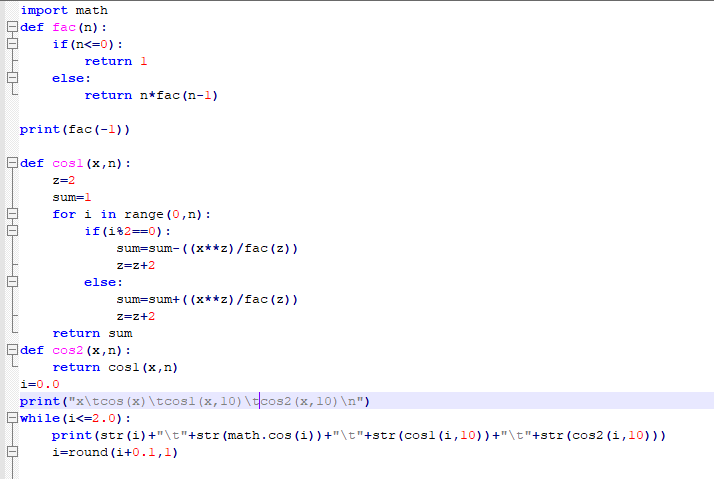#output: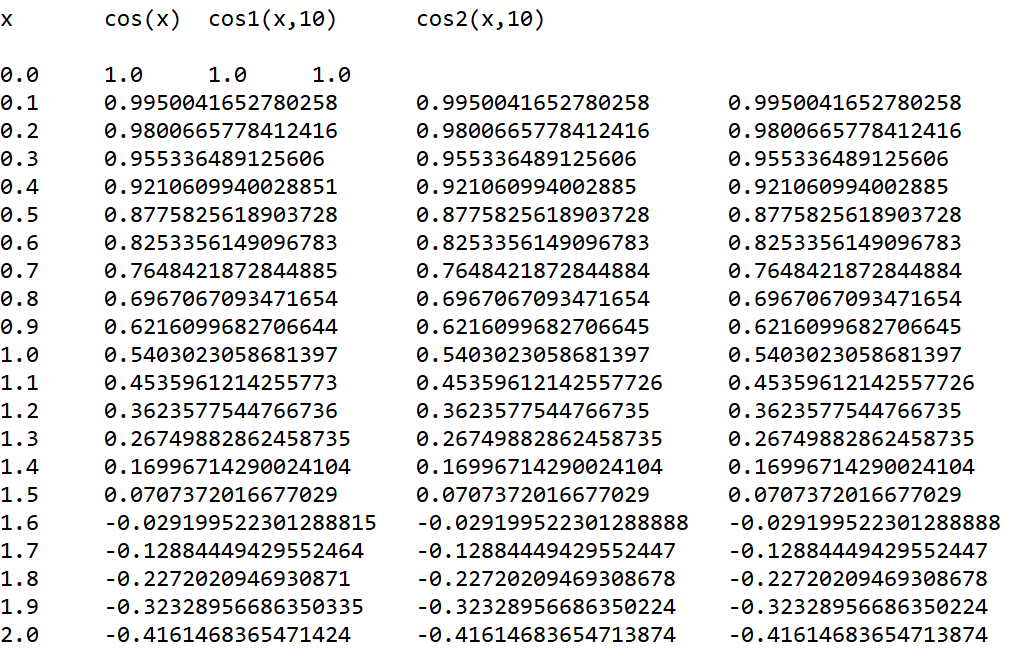#if you have any doubt comment below...

#### Earn Coin

Coins can be redeemed for fabulous gifts.

Similar Homework Help Questions
• ### Evaluate the integral for values of x from 0 to 3 in steps of 0.1 AND plot the integral. The prog...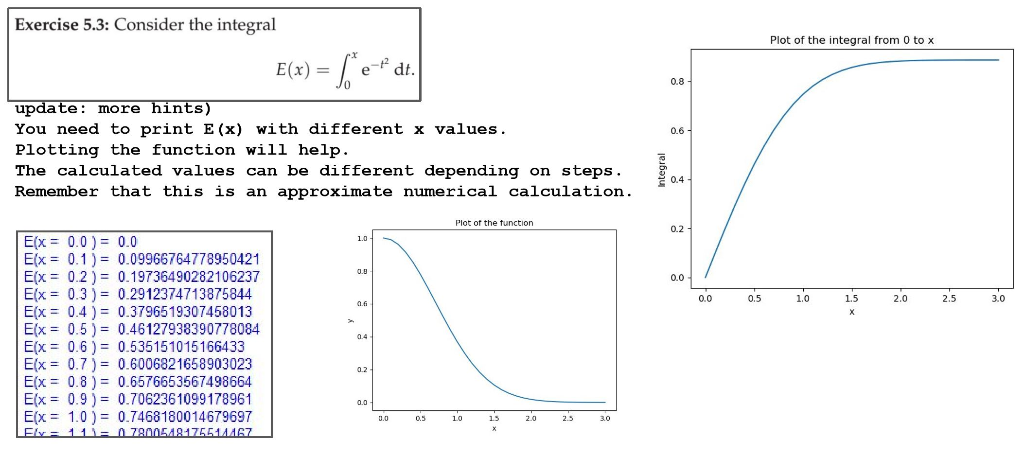Evaluate the integral for values of x from 0 to 3 in steps of 0.1 AND plot the integral. The program needs to be written in Python 3. Can only import numpy and matplotlib. CAN NOT use lambda functions or classes of any kind. Exercise 5.3: Consider the integral Plot of the integral from 0 to x 0.8 e: more hints You need to print E(x) with different x values. 0.6 1 Plotting the function will help The calculated values...

• ### Lab Exercise #11 Assignment Overview You will work with a partner on this exercise during your la...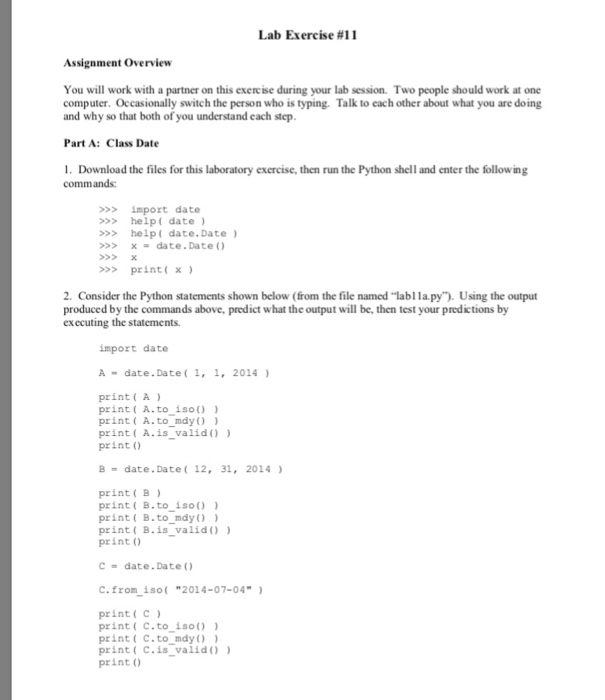help with cse problem Lab Exercise #11 Assignment Overview You will work with a partner on this exercise during your lab session. Two people should work at one computer. Occasionally switch the person who is typing. Talk to each other about what you are doing and why so that both of you understand each step Part A: Class Date . Download the files for this laboratory exercise, then run the Python shell and enter the following commands: >>>import date >>help(...

• ### Please Write in C++ (10-30) @ 11:55pm 1.9 HW7 This homework assignment gives you the opportunity...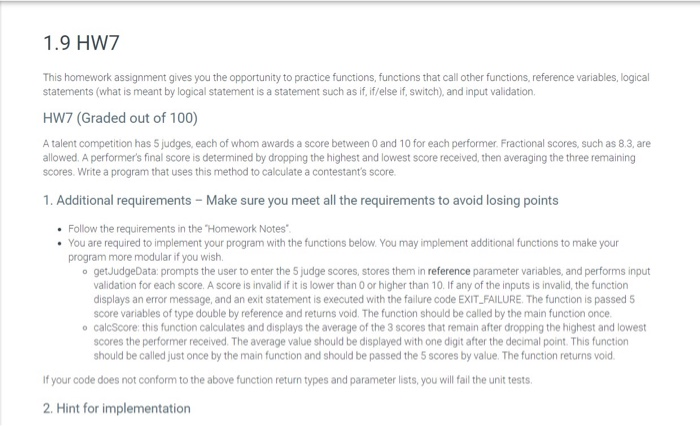Please Write in C++ (10-30) @ 11:55pm 1.9 HW7 This homework assignment gives you the opportunity to practice functions, functions that call other functions, reference variables, logical statements (what is meant by logical statement is a statement such as if, if/else if, switch), and input validation, HW7 (Graded out of 100) A talent competition has 5 judges, each of whom awards a score between 0 and 10 for each performer. Fractional scores, such as 8.3, are allowed. A performer's final...

• ### C++ CODE /* This is program project 2 on page 695. * Before you begin the...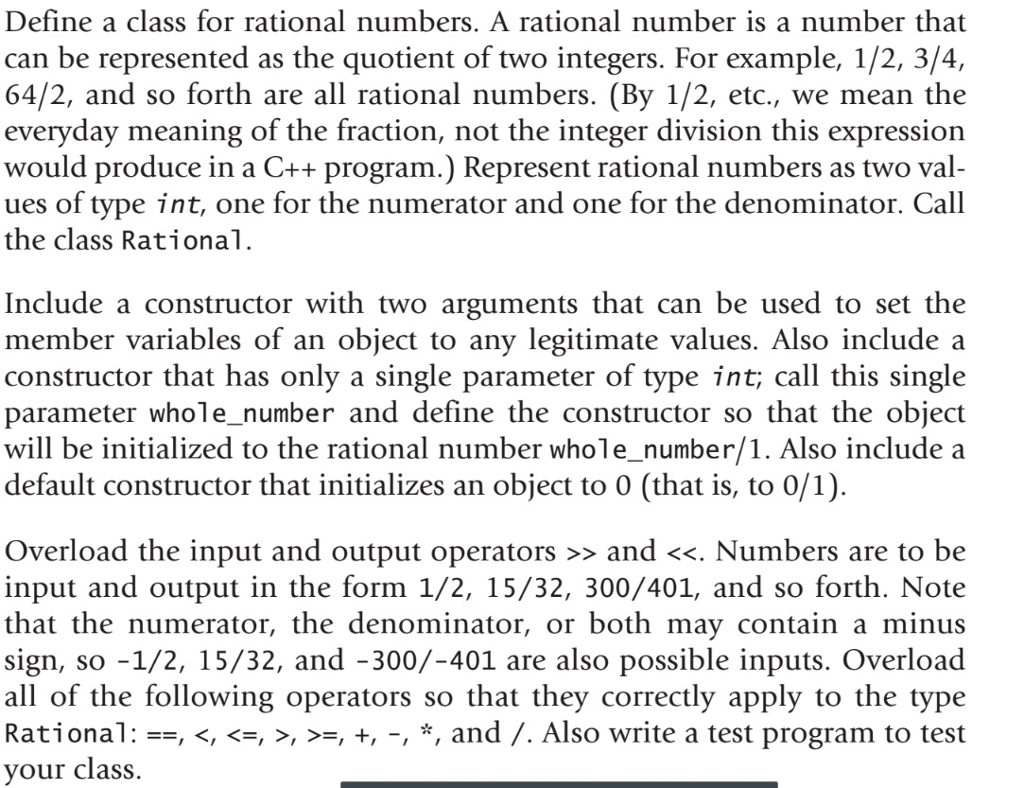C++ CODE /* This is program project 2 on page 695. * Before you begin the project, please read the project description * on page 695 first. * * Author: Your Name * Version: Dates */ #include <iostream> #include <cmath> #include <cassert> using namespace std; class Fraction { public: // constructor Fraction(int a, int b); // generate a fraction which is a/b Fraction(int a); // generate a fraction which is a/1 Fraction(); // generate a fraction which is 0/1. i.e...

• ### use python IDEL Please highlight the answer 1 ווCIוסטIT Errors and Exceptions. There are two main...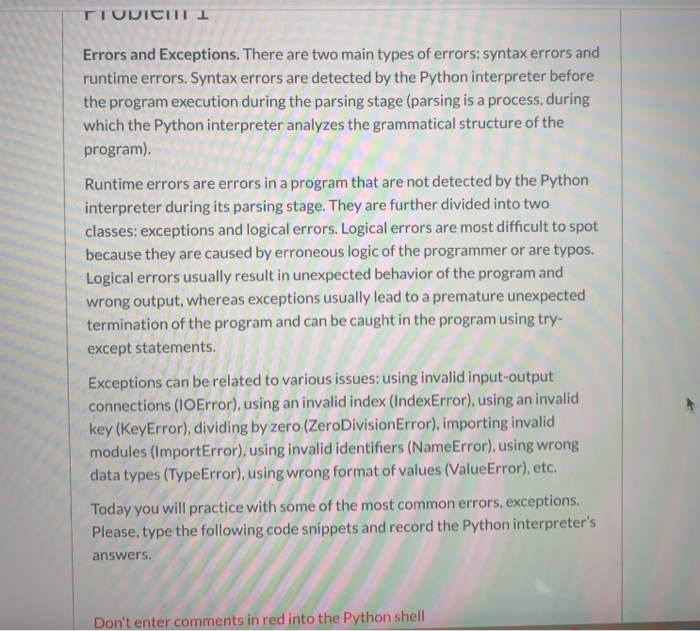use python IDEL Please highlight the answer 1 ווCIוסטIT Errors and Exceptions. There are two main types of errors: syntax errors and runtime errors. Syntax errors are detected by the Python interpreter before the program execution during the parsing stage (parsing is a process, during which the Python interpreter analyzes the grammatical structure of the program). Runtime errors are errors in a program that are not detected by the Python interpreter during its parsing stage. They are further divided into...

• ### C++ CODE /* This is program project 2 on page 695. * Before you begin the...C++ CODE /* This is program project 2 on page 695. * Before you begin the project, please read the project description * on page 695 first. * * Author: Your Name * Version: Dates */ #include <iostream> #include <cmath> #include <cassert> using namespace std; class Fraction { public: // constructor Fraction(int a, int b); // generate a fraction which is a/b Fraction(int a); // generate a fraction which is a/1 Fraction(); // generate a fraction which is 0/1. i.e...

• ### Python 3 Question Please keep the code introductory friendly and include comments. Many thanks. Prompt: The...

Python 3 Question Please keep the code introductory friendly and include comments. Many thanks. Prompt: The owners of the Annan Supermarket would like to have a program that computes the weekly gross pay of their employees. The user will enter an employee’s first name, last name, the hourly rate of pay, and the number of hours worked for the week. In addition, Annan Supermarkets would like the program to compute the employee’s net pay and overtime pay. Overtime hours, any...

• ### python code，please！ Task 3:N ns Brute For In mathematics, the notion of permutation relates to the act of arranging all the members of a set into some sequence or order, or if the set is already or...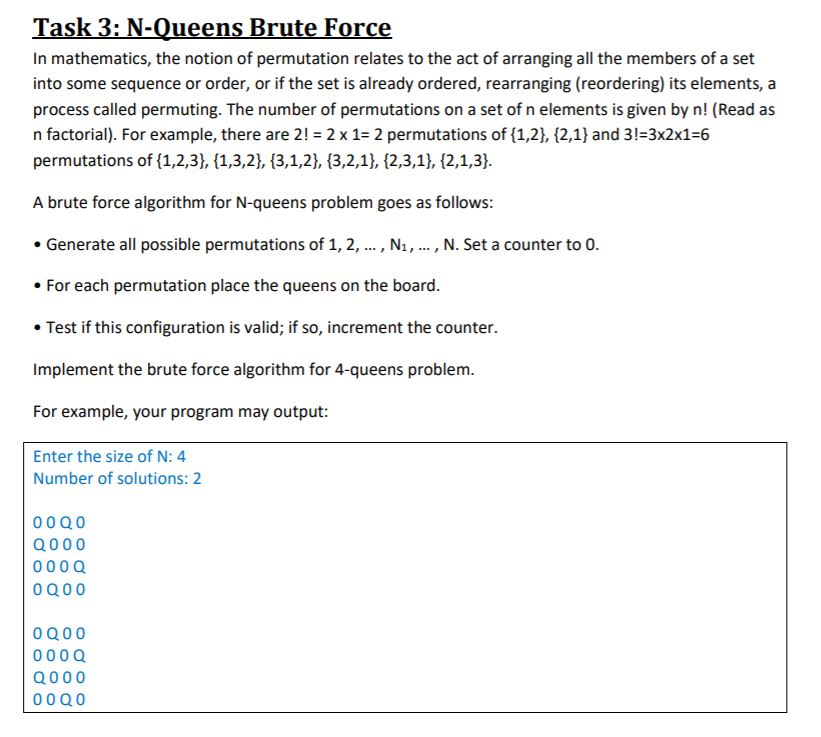python code，please！ Task 3:N ns Brute For In mathematics, the notion of permutation relates to the act of arranging all the members of a set into some sequence or order, or if the set is already ordered, rearranging (reordering) its elements, a process called permuting. The number of permutations on a set of n elements is given by n! (Read as n factorial). For example, there are 2!2 x 1- 2 permutations of 11,2), 2,1) and 3!-3x2x16 permutations of (1,2,3),...

• ### C++ please Programming Assignment #6 Help Me Find The Secret Message Description: This assignment will require...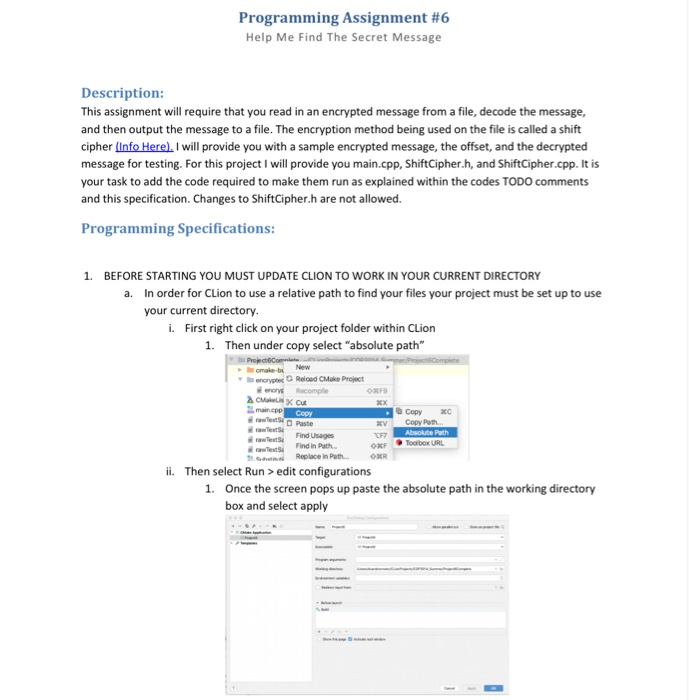C++ please Programming Assignment #6 Help Me Find The Secret Message Description: This assignment will require that you read in an encrypted message from a file, decode the message, and then output the message to a file. The encryption method being used on the file is called a shift cipher (Info Here). I will provide you with a sample encrypted message, the offset, and the decrypted message for testing. For this project I will provide you main.cpp, ShiftCipher.h, and ShiftCipher.cpp....

• ### please show work!! Additional Algo 14-3 Standard Deviation of Demand Over Multiple Periods Each period, demand...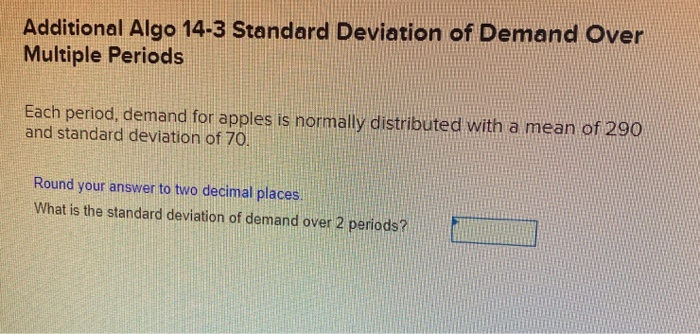please show work!! Additional Algo 14-3 Standard Deviation of Demand Over Multiple Periods Each period, demand for apples is normally distributed with a mean of 290 and standard deviation of 70. Round your answer to two decimal places. What is the standard deviation of demand over 2 periods? Additional Algo 14-1 Inventory Position For a particular item, a firm has established an order-up-to level of 235 units. Currently, there are 65 units in stock, O backorders and 75 units scheduled...